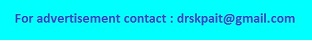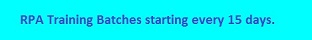Buoyant Force Calculator# Buoyancy Force Calculation

The calculator will calculate the buoyant force exerted by liquid when an object is immersed inside the liquid for given values of density of fluid and displaced volume. Objects appear to be less heavy in water/liquid because the water/liquid exerts an upward force on the objects immersed in it
Formula: Buoyancy Force (B) = ρ V g
Where,     ρ = Density of Fluid,     V = Displaced Volume,     g = 9.8 (gravity).

 Density of Fluid (ρ) = kg m-3 None Peta Tera Giga Mega Kilo Centi Milli Micro Nano Pico Femto Displaced Volume (V) = kg m-3 None Peta Tera Giga Mega Kilo Centi Milli Micro Nano Pico Femto Buoyant Force (B) = Newton
 Buoyancy Force is an upward force exerted by a fluid that opposes the weight of an immersed object and the pressure increases as the depth increases. When any object is placed in a fluid then fluid exerts an upward force we call the buoyant force, buoyancy force is present whether the object floats or sinks, the pressure on the bottom of an object is always larger than the force on the top.